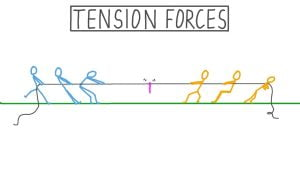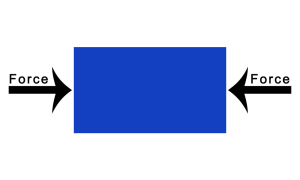Monday, October 2, 2023

# Difference Between Tension and Compression Force

In this article, we will discuss the difference between tension and compression force.

### 1. Introduction

#### a. Tension Force

Tension is a force that simply stretches the material.

Commonly it is transferred through a rope, string, or a wire when pulled by forces acting from opposite sides.

The tension force is placed over the whole length of the wire and pulls equally on the body at the ends.Examples of Tension Force.

1. Two men pulling a rope

2. A car towing another car with the help of a chain.

3. Crane machine

4. Pulling a bucket of water from the well.

The Formula of Tension.

T = Mg

Where,

T= Tension Force

M = Mass (kg)

g= Gravitational Force = 9.2 m/s²

#### b. Compression Force

An internal force that compacts or squeezes the particles of an object is known as compression force. Its SI unit is Newton. The product of mass and Area of the body results in compression force.

For example:

The springs in a mattress compact together when you sleep down on the bed.  Objects, when compressed, generally return back to their original shape once the external force is lifted.

Another example consists of kicking a soccer ball and stepping with your shoe.The Formula of Compression.

N = M x a

N= Compression Force

M = Mass (kg)

a = Acceleration due to gravity = 9.8m/s²

Compressive force per unit area is known as compressive stress.

And the compressive strain is the ratio of reduced length to the original length.

### 2. Difference Between Tension and Compression Force

 S.no Tension force Compression force 1. It is considered as Force. It is considered a Phenomenon. 2. A tension force is a force that tends to pulls materials apart. A compression force is a force that tends to squeezes material. 3. The force that tends to increase the length of a body or an object is called tension. The force that tends to compact the body or an object is called compression. 4. The forces acting upon the body are directed away from the object. The forces acting upon the body are directed toward the body. 5. Related to object: can be related to pulling on the ends of a rod. Related to object: can be associated with pushing on the ends of a rod toward the middle. 6. Applicable: only applied in solid strings. Applicable: can be valid to any material. 7. Examples of Tension Force: Ropes, the cable of crane, threads, etc. Examples of Compression Force: Concrete pillars, steel pillars, etc.

### 3. QNA

What is Compression Force?

An internal force that compacts or squeezes the particles of an object.

What are examples of compression force?

Concrete pillars, kicking a soccer ball and stepping with your shoes, etc are examples of compression force.

What is Tension force?

Tension is a force that generally stretches something.

What are examples of Tension force?

Ropes, cables of cranes, threads, etc are examples of Tension Force.

 Read More:  Tension Test on Steel Rod

 Read More: Compression test on brickHe is a founder and lead author of Dream Civil International and his civil engineering research articles has been taken as source by world's top news and educational sites like USA Today, Time, The richest, Wikipedia, etc.
Latest Articles

Related Articles